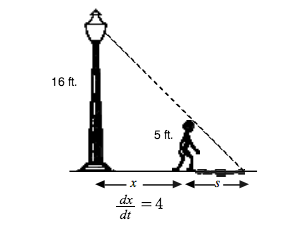### Home > APCALC > Chapter 7 > Lesson 7.2.2 > Problem7-66

7-66.

Remember Eric and the $16$-foot tall lamppost? If Eric (who is $5$ feet tall) walks away from the lamppost at a rate of $4$ ft/sec, at what rate is the tip of his shadow moving away from the lamppost? Draw a diagram before you start this problem.Use similar triangles to write an equation relating the proportional sides.

Use implicit differentiation to convert the geometric equation into a rate equation.
In other words, find the derivative of everything in terms of time, $t$.